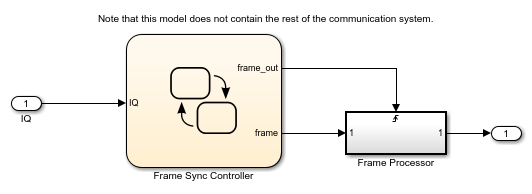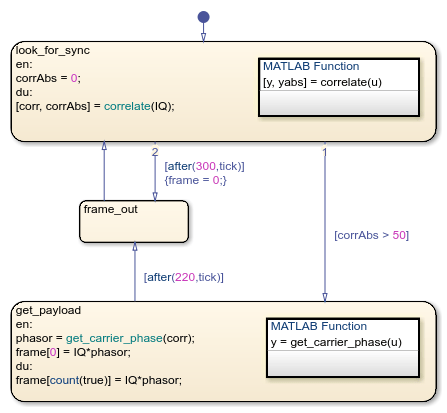# Detect Valid Transmission Data by Using Frame Synchronization

This example shows how to process complex data in a Stateflow® chart. The model uses vectors of complex data to find a fixed pattern in a signal from a communication system.

### Frame Synchronization

In communication systems, frame synchronization is a method of finding valid data in a transmission that consists of data frames. To aid frame synchronization, the transmitter inserts a fixed data pattern at the start of each data frame to mark the start of valid data. The receiver searches for the fixed pattern and achieves frame synchronization when the correlation between the input data and the fixed pattern is high.In this example, the Stateflow chart Frame Sync Controller accepts a complex input signal `IQ` and searches for the fixed data pattern `trainSig`. After recognizing the start of a data frame, the chart stores the valid data in a complex output signal `frame`. This output signal is a vector of complex products between each valid data point and the phase angle of the carrier wave. The chart then passes the valid data to the Frame Processor subsystem.Note that this model does not contain the rest of the communication system.

### Synchronize Data Frame

In the Frame Sync Controller chart, the `look_for_sync` state starts the frame synchronization algorithm. At each time step, the MATLAB® function `correlate` calculates the correlation between the input signal `IQ` and the fixed data pattern `trainSig`. The function stores the complex correlation as `corr` and its absolute value as `corrAbs`. The value of `corrAbs` is the correlation percentage, which can range from 0 to 100 percent. At 0 percent, there is no correlation. At 100 percent, there is perfect correlation.

• If `corrAbs` exceeds 50 percent, the correlation is high and the chart registers the start of a data frame. The chart takes the transition to the `get_payload` state and stores 220 valid data points in the complex vector `frame`.

• If `corrAbs` stays below 50 percent for 300 consecutive data points, the frame synchronization algorithm resets. The chart takes the transition to the `frame_out` state and triggers the Frame Processor subsystem. The chart then returns to the `look_for_sync` state.

### Store Scalar Data in a Vector

When the Frame Sync Controller chart recognizes the start of a data frame, the `get_payload` state calls the MATLAB function `get_carrier_phase` to compute the phase angle of the carrier wave. The state stores this phase angle as the local data object `phasor`. Then the state collects the scalar values of the product of `IQ*phasor` in the vector `frame`. To avoid using an extra variable as an index counter, this state indexes into this vector by using the `count` operator:

• When the state becomes active, the `entry` action stores the initial value of the product in the first element of `frame`.

• In later time steps, the `during` action stores the next values of this product in successive elements of `frame`. The indexing expression `count(true)` returns the number of time steps since the state became active.

After 220 times steps, the transition condition `[after(220,tick)]` becomes `true` and the chart exits from the state. When the chart enters the `frame_out` state, the vector `frame` contains the values of 220 products. The chart passes this data to the Frame Processor subsystem and then returns to the `look_for_sync` state.